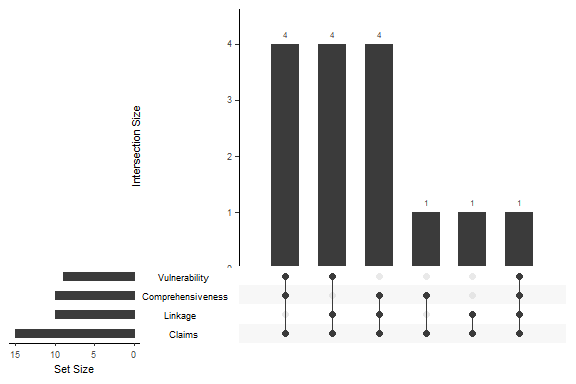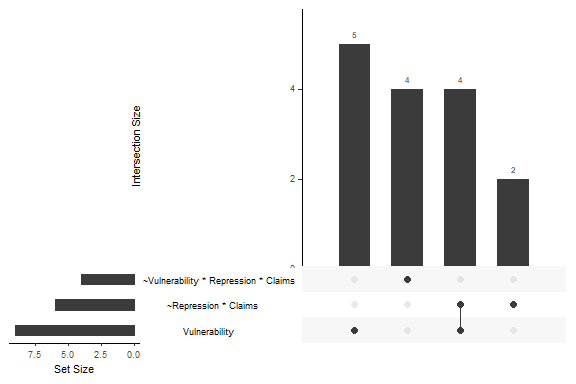# Aggregation over partitions

#### 2021-10-26

library(QCAcluster)

The functions upset_conditions() and upset_conjunctions() can be used after one has derived partition-specific models with partition_min() or partition_min_inter(). The functions take the models from the solution column of the dataframes produced with partition_min()/or partition_min_inter() as input to produce an UpSet plot. upset_conditions() and upset_configurations() are functions that draw on the upset() function of the UpSetR package. We use the dataset by Grauvogel and von Soest (2014) for illustrating the meaning and interpretation of UpSet plots.

### Aggregation over individual conditions

The following plot aggregates the occurrence and co-occurrence of single conditions over the partition-specific parsimonious solutions.

data("Grauvogel2014")
# parsimonious solution for each type of Sender
GS_pars <- partition_min(
dataset = Grauvogel2014,
units = "Sender",
"Repression", "Claims"),
out = "Persistence",
n_cut = 1, incl_cut = 0.75,
solution = "P",
BE_cons = rep(0.75, 3),
BE_ncut = rep(1, 3))
# UpSet plot with three sets
upset_conditions(GS_pars, nsets = 4)Each line (or horizontal bar) in an UpSet plot displays how often a single condition occurs over all 16 partition-specific models. The plot shows that Claims occurs as a single condition in 15 models out of 16.

Each column or vertical bar in the plot shows how often single conditions occur together in a model. (This means two conditions that co-occur in a model are not necessarily part of the same conjunction.) The plot shows that three different sets of conditions occur together in five models each and that three more combinations of conditions are specific to one model each.

### Aggregation over individual sufficient terms

The function upset_configurations() decomposes models into the constitutive sufficient terms. A ‘term’ can be a configuration (aka as conjunction) of conditions or single sufficient conditions. Nonetheless, we called this function upset_configurations() for convenience and to distinguish it from the upset_conditions() function.

upset_configurations(GS_pars, nsets = 3)Over one pooled model and 15 within-models (there is extensive model ambiguity), the individually sufficient condition Vulnerability is part of eight models and is the only sufficient term in five models. In three additional models, it coincides with the conjunction ~Repression * Claims. In total, ~Repression * Claims occurs six times in the 16 models.